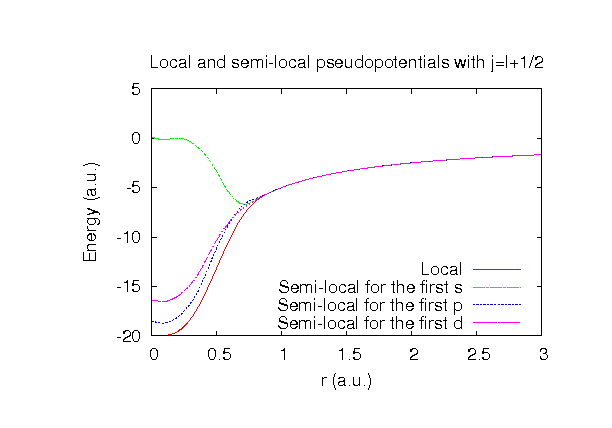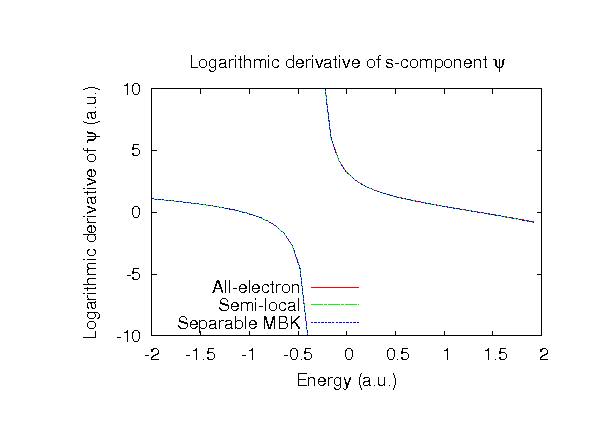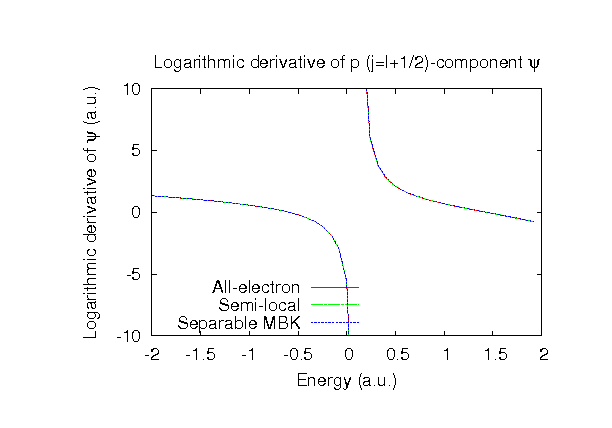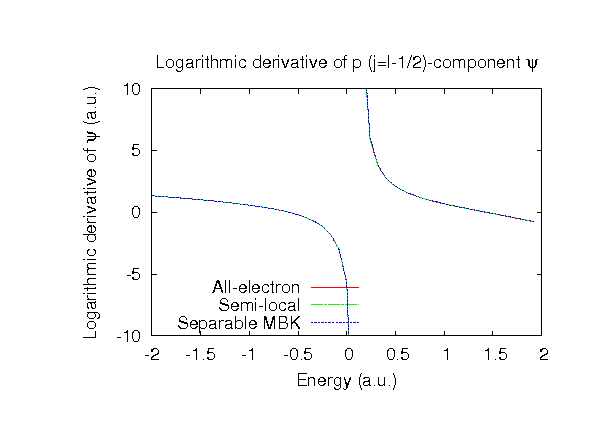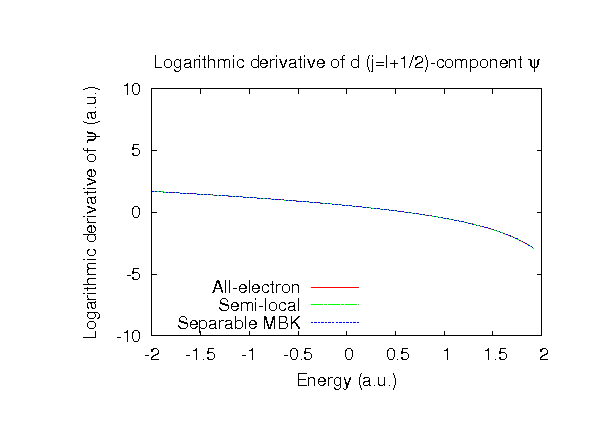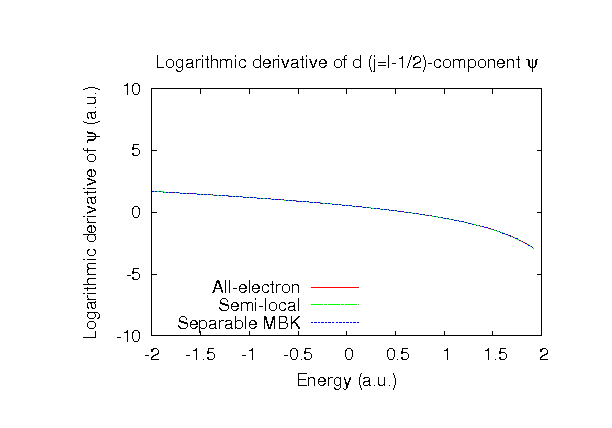### Fully relativistic pseudopotentials

Fully relativistic pseudopotentials generated by the MBK (PRB 47, 6728 (1993)) scheme within LDA (CA13) and GGA (PBE13) which contain a partial core correction and fully relativistic effects including spin-orbit coupling.

### Pseudo-atomic orbitals

The number below the symbol means a cutoff radius (a.u.) of the confinement potential. These file includes fifteen radial parts for each angular momentum quantum number l (=0,1,2,3). The basis functions were generated by variationally optimizing the corresponding primitive basis functions in the single atom and the dimer molecule. The input files used for the orbital optimization can be found at N_opt.dat , and N2_opt.dat . Since N_CA13.vps and N_PBE13.vps include the 2s and 2p states (5 electrons) as the valence states, the minimal basis set is N*.*-s1p1. Our recommendation for the choice of cutoff radius of basis functions is that N5.0.pao is enough for bulks, but N6.0.pao or N7.0.pao is preferable for molecular systems.

### Benchmark calculations by the PBE13 pseudopotential with the various basis functions

(1) Calculation of the total energy as a function of lattice constant in the bcc structure, where the total energy is plotted relative to the minimum energy for each case. a0 and B0 are the equilibrium lattice constant and bulk modulus obtained by fitting to the Murnaghan equation of state. The difference between N6.0-s2p2d1 and N6.0-s3p3d2 (N6.0-s3p3d2f1) in the total energy at the minimum point is 0.067 (0.086) eV/atom. An input file used for the OpenMX calculations can be found at Nbcc-EvsV.dat . For comparison the result by the Wien2k code is also shown, where the calculation was performed by default setting in the Ver. 10.1 of Wien2k except for the use of RMT x KMAX of 12.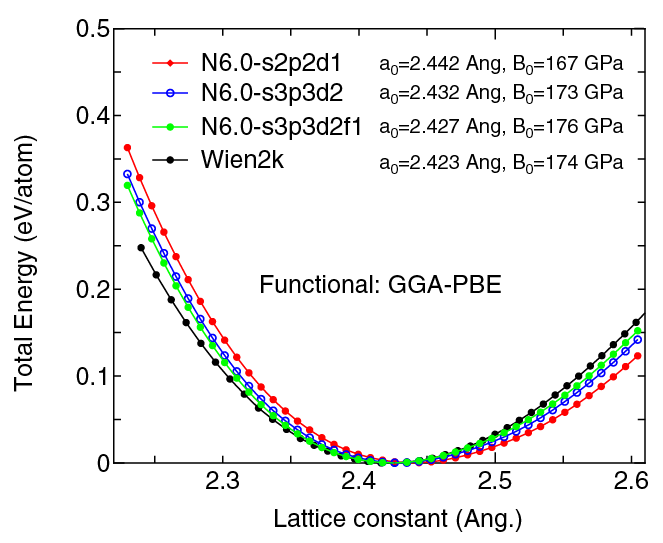(2) Calculations of the band dispersion in the bcc structure, where the non-spin polarized collinear calculation with the lattice constant of 2.43 Ang. was performed using N_PBE13.vps, N6.0-s2p2d1, and N6.0-s3p3d2, and the origin of the energy is taken to be the Fermi level. The input file used for the OpenMX calculations can be found at Nbcc-Band.dat . For comparison the result by the Wien2k code is also shown, where the calculation was performed by default setting in the Ver. 10.1 of Wien2k except for the use of RMT x KMAX of 12.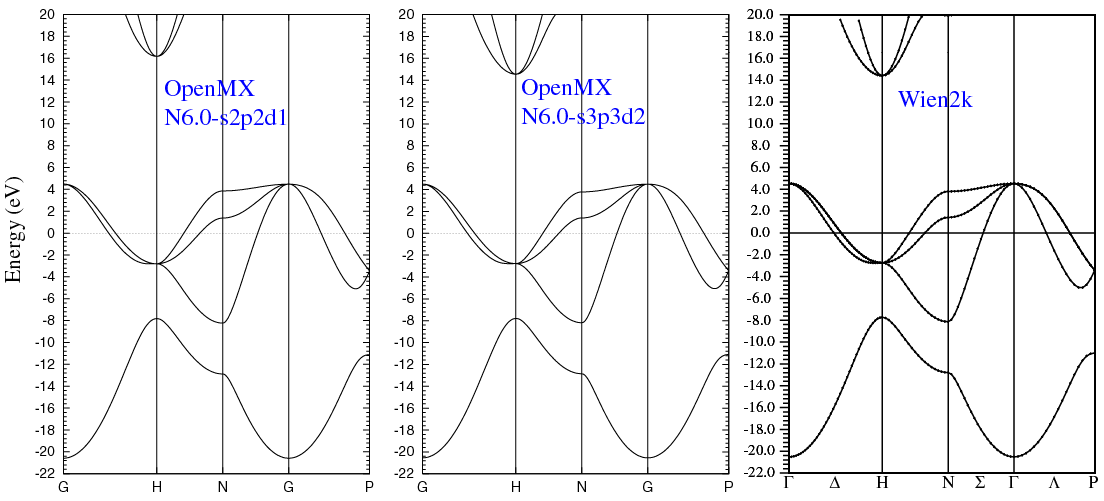(3) Calculations of a N2 molecule, where N_PBE13.vps, N7.0-s2p2d1, N7.0-s3p3d2, and N7.0-s3p3d2f1 were used. The input files used for the OpenMX calculations can be found at N2.dat , N_cp1.dat , N_cp2.dat , N_cp3.dat , and N.dat .

 Equilibrium bond length (Ang.) Atomization energy (kcal/mol) Atomization energy (couterpoise corrected) (kcal/mol) N7.0-s2p2d1 1.119 221.9 221.7 N7.0-s3p3d2 1.118 223.0 222.7 N7.0-s3p3d2f1 1.117 223.2 222.9 Other calc. 1.111 a 236.7a Expt. 1.098b 227b

a M.M. Odashima and and K. Capelle, J. Chem. Theory Comput. 5 798 (2009).
b Taken from M. Ernzerhof and G.E. Scuseria, J. Chem. Phys. 110, 5029 (1999).

### Supplementary information for the GGA (PBE13) pseudopotential